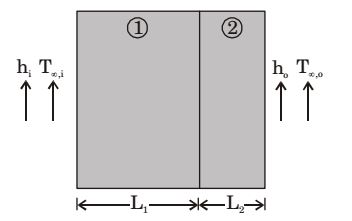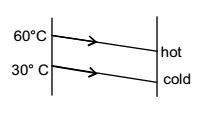## Heat Transfer Miscellaneous

#### Heat-Transfer

1. Biot number signifies the ratio of

1. Biot - number:
Biot number provides a way to compare the conduction resistance within a solid body to the convection resistance external to the body (offered by the surrounding fluid) for heat transfer:

 Bi = hs ; s = Volume of the body k Surface area

Where ‘s’ is a characteristic dimension of the solid
‘h’ is convective heat transfer coefficient
‘k’ is thermal conductivity of the body.

##### Correct Option: B

Biot - number:
Biot number provides a way to compare the conduction resistance within a solid body to the convection resistance external to the body (offered by the surrounding fluid) for heat transfer:

 Bi = hs ; s = Volume of the body k Surface area

Where ‘s’ is a characteristic dimension of the solid
‘h’ is convective heat transfer coefficient
‘k’ is thermal conductivity of the body.

1. A steel ball of diameter 60 mm is initially in thermal equilibrium at 1030°C in a furnace. It is suddenly removed from the furnace and cooled in ambient air at 30°C, with convective heat transfer coefficient h = 20 W/m²K. The thermophysical properties of steel are: density ρ = 7800 kg/m², conductivity k = 40 W/mK and specific heat c - 600 J/kgK. The time required in seconds to cool the steel ball in air from 1030°C to 430°C is

1. Diameter of steel ball,
d = 0.06 m
Initial temperature,
Ti = 1030°C
Ambient temperature
T0 = 30°C
Convective heat transfer coefficient

 h = 20 W m²K

 ρ = 7800 kg m³

 k = 40 W mK

 C = 600 J kg

Final temperature of steel ball, T = 430°c
Time: t =?
 Ti - T0 = e-ht/ρCl.......(i) Ti - T0

 l = V = πd³ = d = 0.6 = 0.1 m A 6 × πd² 6 6

From (i)
 430 - 30 = e-20×t/7800×600×0.1 1030 - 30

t = 2144.16 seconds

##### Correct Option: D

Diameter of steel ball,
d = 0.06 m
Initial temperature,
Ti = 1030°C
Ambient temperature
T0 = 30°C
Convective heat transfer coefficient

 h = 20 W m²K

 ρ = 7800 kg m³

 k = 40 W mK

 C = 600 J kg

Final temperature of steel ball, T = 430°c
Time: t =?
 Ti - T0 = e-ht/ρCl.......(i) Ti - T0

 l = V = πd³ = d = 0.6 = 0.1 m A 6 × πd² 6 6

From (i)
 430 - 30 = e-20×t/7800×600×0.1 1030 - 30

t = 2144.16 seconds

1. Consider steady-state heat conduction across the thickness in a plane composite wall (as shown in the figure) exposed to convection conditions on both sides.
Given: hi = 20 W/m²K; h0 = 50 W/m²K; T∞i = 20°C; T*infin;,0 = –2°C; k1 = 20 W/mK; k2 = 50 W/mK; L1 = 0.30 m and L2 = 0.15 m.
Assuming negligible contact resistance between the wall surfaces, the interface termperature, T(in °C), of the two walls will be1.  Req = 1 + l1 + l2 + 1 h2A h1A h2A h2A

 = 11 + 0.30 + 0.15 + 1= 0.088 A 20 20 50 50 A

 Now Q2 = (t1 + t2) = t1 - t Req 1 + L1 hiA h1A

 ∴ t1 - t = t1 - t 0.8 0.065 A A

 ⇒ 2.95 × 0.065 = 293 - t 0.088

⇒ t = 3.75°C
Alternately
 (20 - ti) = t1 - (-2) 1 + l1 l2 + 1 hiA k1A k2A h0A

 ∴ 20 - ti = t1 + 2 1 + 0.3 0.15 + 1 20 20 50 50

 or 20 - ti = T1 + 2 1.3 1.15 20 50

 or 20 - ti = 1.3 × 50 {ti + 2} 1.05 20

or 20 – ti = 2.826 (ti + 2)
or 3.826 ti = 20 – 2 × 2.826
or ti = 3.75°C

##### Correct Option: C

 Req = 1 + l1 + l2 + 1 h2A h1A h2A h2A

 = 11 + 0.30 + 0.15 + 1= 0.088 A 20 20 50 50 A

 Now Q2 = (t1 + t2) = t1 - t Req 1 + L1 hiA h1A

 ∴ t1 - t = t1 - t 0.8 0.065 A A

 ⇒ 2.95 × 0.065 = 293 - t 0.088

⇒ t = 3.75°C
Alternately
 (20 - ti) = t1 - (-2) 1 + l1 l2 + 1 hiA k1A k2A h0A

 ∴ 20 - ti = t1 + 2 1 + 0.3 0.15 + 1 20 20 50 50

 or 20 - ti = T1 + 2 1.3 1.15 20 50

 or 20 - ti = 1.3 × 50 {ti + 2} 1.05 20

or 20 – ti = 2.826 (ti + 2)
or 3.826 ti = 20 – 2 × 2.826
or ti = 3.75°C

1. The logarithmic mean temperature difference (LMTD) of a counter flow heat exchanger is 20°C. The cold fluid enters at 20°C and the hot fluid enters at 100°C. Mass flow rate of the cold fluid is twice that of the hot fluid. Specific heat at constant pressure of the hot fluid is twice that of the cold fluid. The exit temperature of the cold fluid

1. θ1 = θ2
θm = 20°C
Th1 – Tc2 = 20°C
100 – Tc2 = 20°C
Tc2 = 80°C

##### Correct Option: C

θ1 = θ2
θm = 20°C
Th1 – Tc2 = 20°C
100 – Tc2 = 20°C
Tc2 = 80°C

1. In a counterflow heat exchanger, hot fluid enters at 60°C and cold fluid leaves at 30°C. Mass flow rate of the hot fluid is 1 kg/s and that the cold fluid is 2 kg/s. Specific heat of the hot fluid is 10 kJ/kgK and that of the cold fluid is 5 kJ/kgK. The Log Mean Temperature Difference (LMTD) for the heat exchanger in °C is

1. Heat capacity of hot fluid
= 1 × 10 = 10 kJ/ k – s
Heat capacity of cold fluid
= 2 × 5 = 10 kJ/ k – s
Since heat capacity is same, so LMTD is difference of temperature at either end
i.e. LMTD = 60° – 30° = 30° C##### Correct Option: B

Heat capacity of hot fluid
= 1 × 10 = 10 kJ/ k – s
Heat capacity of cold fluid
= 2 × 5 = 10 kJ/ k – s
Since heat capacity is same, so LMTD is difference of temperature at either end
i.e. LMTD = 60° – 30° = 30° C